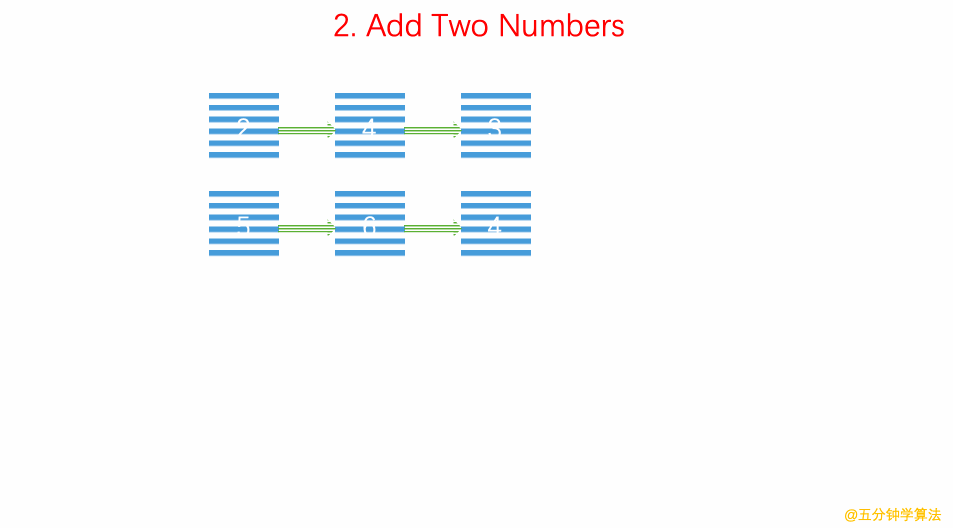# LeetCode 第 2 号问题：两数相加

### 题目描述

``````输入：(2 -> 4 -> 3) + (5 -> 6 -> 4)

``````

### 动画描述### 代码实现

``````/// 时间复杂度: O(n)
/// 空间复杂度: O(n)
class Solution {
public:
ListNode* addTwoNumbers(ListNode* l1, ListNode* l2) {

ListNode *p1 = l1, *p2 = l2;
ListNode *dummyHead = new ListNode(-1);
ListNode* cur = dummyHead;
int carried = 0;
while(p1 || p2 ){
int a = p1 ? p1->val : 0;
int b = p2 ? p2->val : 0;
cur->next = new ListNode((a + b + carried) % 10);
carried = (a + b + carried) / 10;

cur = cur->next;
p1 = p1 ? p1->next : NULL;
p2 = p2 ? p2->next : NULL;
}

cur->next = carried ? new ListNode(1) : NULL;
ListNode* ret = dummyHead->next;
delete dummyHead;
return ret;
}
};

``````